Jet Set Go! All about Aeroplanes Jet Set Go! All about Aeroplanes

# Symmetry And Line Of Symmetry: An Introduction

In geometry, symmetry is defined as a balanced and proportionate similarity that is found in two halves of an object. It means one-half is the mirror image of the other half. The imaginary line or axis along which you can fold a figure to obtain the symmetrical halves is called the line of symmetry.

If an object is symmetrical, it means that it is equal on both sides. Suppose, if we fold a paper such that half of the paper coincides with the other half of the paper, then the paper has symmetry.

Symmetry can be defined for both regular and irregular shapes. For example, a square is a regular (all sides are equal) and a rectangle is an irregular shape (since only opposite sides are equal). The symmetries for both shapes are different. Check different figures with symmetry here.

## Symmetry in Mathematics

In Mathematics, a meaning of symmetry defines that one shape is exactly like the other shape when it is moved, rotated, or flipped. Consider an example, when you are told to cut out a ‘heart’ from a piece of paper, don’t you simply fold the paper, draw one-half of the heart at the fold and cut it out to find that the other half exactly matches the first half? The heart carved out is an example of symmetry.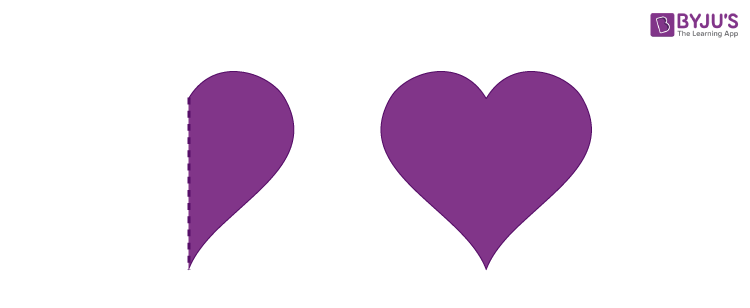Symmetry Math definition states that “symmetry is a mirror image”. When an image looks identical to the original image after the shape is being turned or flipped, then it is called symmetry. It exists in patterns. You may have often heard of the term ‘symmetry’ in day to day life. It is a balanced and proportionate similarity found in two halves of an object, that is, one-half is the mirror image of the other half. And a shape that is not symmetrical is referred to as asymmetrical. Symmetric objects are found all around us, in nature, architecture, and art.

### Symmetrical Figures

Symmetrical shapes or figures are the objects where we can place a line such that the images on both sides of the line mirror each other.  The below set of figures form symmetrical shapes when we place a plane or draw the lines.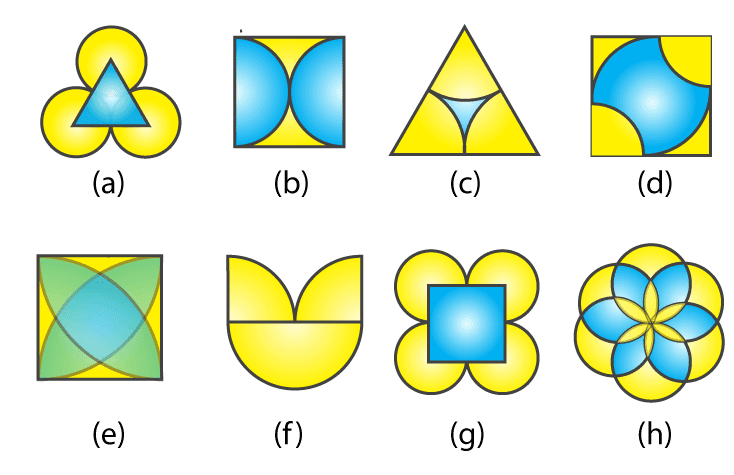For example, figure (b) has the symmetrical figures when we draw two lines of symmetry as shown below.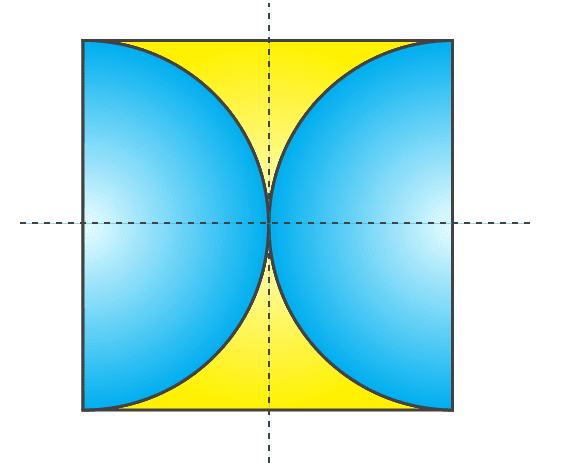## Line of Symmetry

The imaginary line or axis along which you fold a figure to obtain the symmetrical halves is called the line of symmetry. It basically divides an object into two mirror-image halves. The line of symmetry can be vertical, horizontal or diagonal. There may be one or more lines of symmetry.

### 1 Line Symmetry

Figure is symmetrical only about one axis. It may be horizontal or vertical. The word ATOYOTA has one axis of symmetry along the axis passing through Y.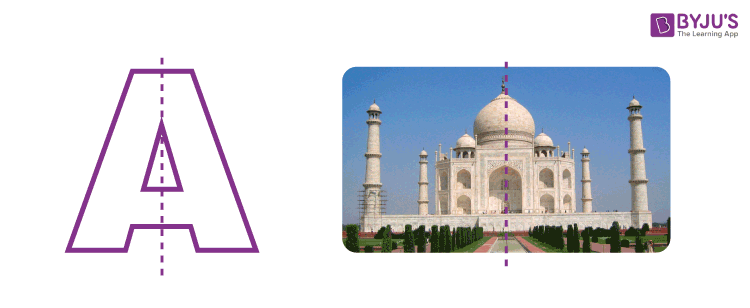### 2 Lines Symmetry

Figure is symmetrical with only about two lines. The lines may be vertical and horizontal lines as viewed in the letters H and X. Thus, we can see here two lines symmetry.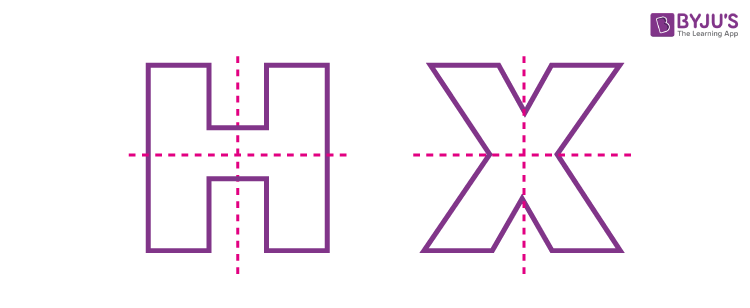### 3 Lines Symmetry

An example of three lines of symmetry is an equilateral triangle. Here, the mirror line passes from the vertex to the opposite side dividing the triangle into two equal right triangles.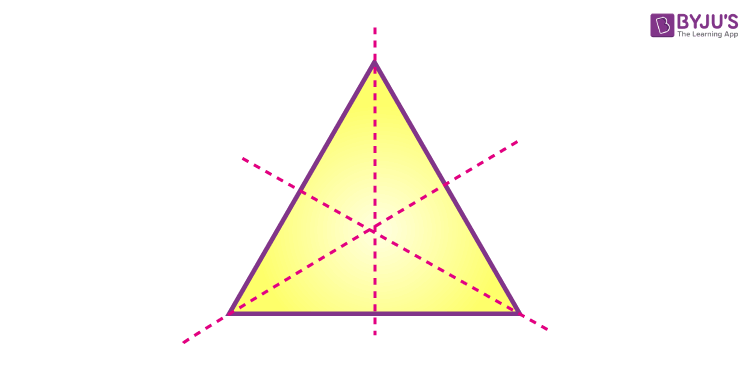### 4 Lines Symmetry

Four lines of symmetry can be seen in a square, that has all the sides equal.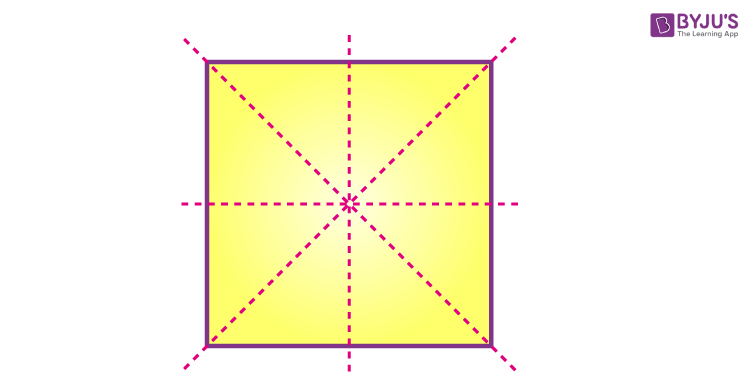### Infinite lines

Some figures have not one or two, but infinite lines passing through the centre, and the figure is still symmetrical. Example: a circle.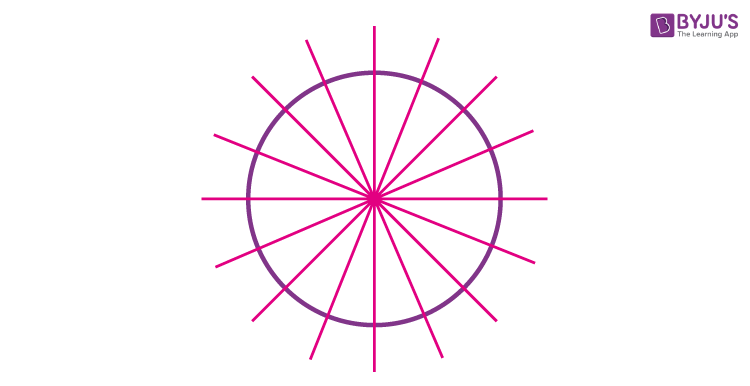### Lines of Symmetry for Different Shapes

Learn below the number of lines of symmetry for different shapes.

## Types of Symmetry

Symmetry may be viewed when you flip, slide or turn an object. There are four types of symmetry that can be observed in various situations, they are:

• Translation Symmetry
• Rotational Symmetry
• Reflection Symmetry
• Glide Symmetry

### Translation Symmetry

If the object is translated or moved from one position to another, the same orientation in the forward and backward motion is called translational symmetry. In other words, it is defined as the sliding of an object about an axis. This can be observed clearly from the figure given below, where the shape is moved forward and backward in the same orientation by keeping the fixed axis.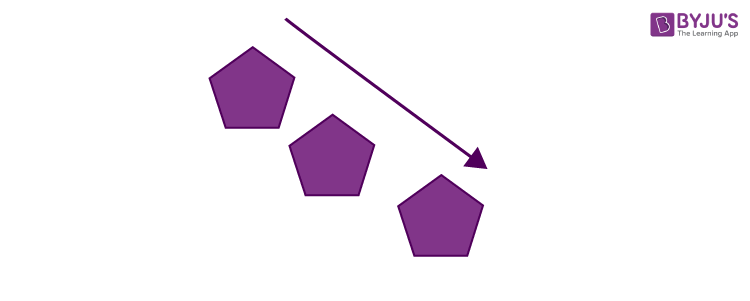### Rotational Symmetry

When an object is rotated in a particular direction, around a point, then it is known as rotational symmetry or radial symmetry. Rotational symmetry existed when a shape turned, and the shape is identical to the origin. The angle of rotational symmetry is the smallest angle at which the figure can be rotated to coincide with itself. The order of symmetry is how the object coincides with itself when it is in rotation.

In geometry, many shapes consist of rotational symmetry. For example, the figures such as circle, square, rectangle have rotational symmetry. Rotational symmetry can also be found in nature, for instance, in the petals of a flower.

Below figure shows the rotational symmetry of a square along with the degree of rotation.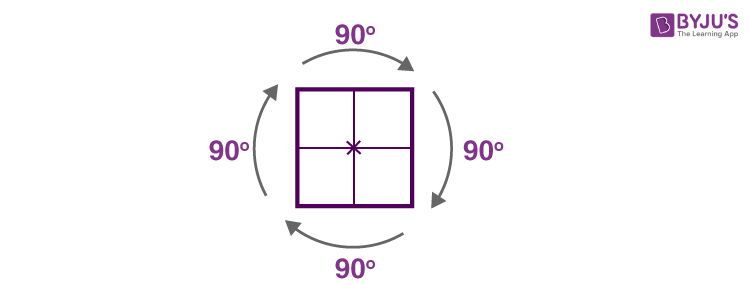### Reflexive Symmetry

Reflection symmetry is a type of symmetry in which one half of the object reflects the other half of the object. It is also called mirror symmetry or line of symmetry. A classic example of reflection symmetry can be observed in nature, as represented in the below figure.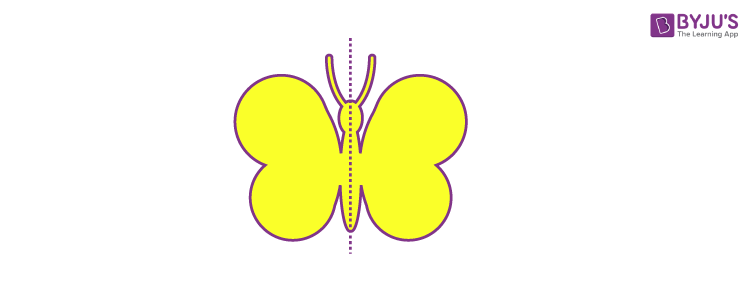### Glide Symmetry

The combination of both translation and reflection transformations is defined as the glide reflection. A glide reflection is commutative in nature. If we change the combination’s order, it will not alter the output of the glide reflection.

## Symmetrical Shapes

The symmetry of shapes can be identified whether it is a line of symmetry, reflection or rotational based on the appearance of the shape.

The shapes can be regular or irregular. Based on their regularity, the shapes can have symmetry in different ways.  Also, it is possible that some shapes does not have symmetry. For example, a tree may or may not have symmetry.

These can be better understood with the examples given below.

### Solved Examples

Example 1: If the figure below follows a reflexive or line of symmetry, then complete the figure.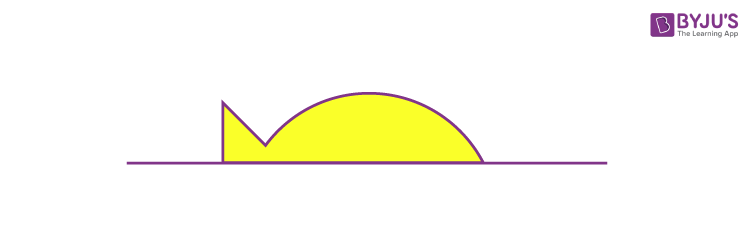Solution:

Given that, the figure has a line of symmetry.

That means, the second half (i.e. missing part) of the figure will be exactly the same as the given.

Thus, the complete figure is: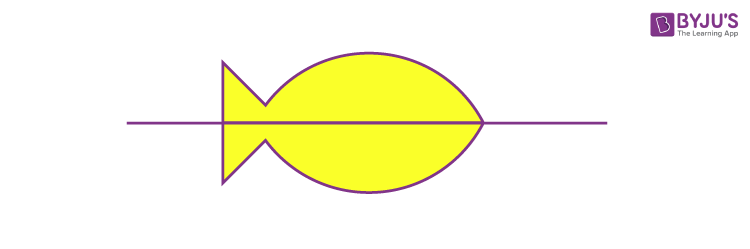Example 2: Identify the shapes which do not have rotational symmetry from the below figure.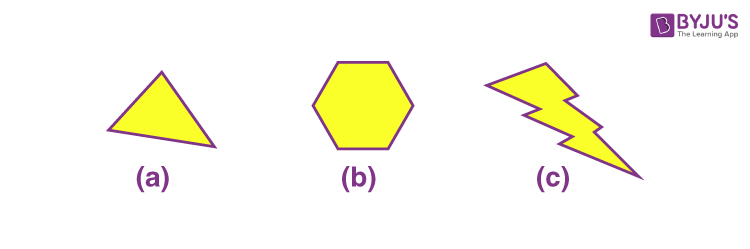Solution:

As we know, rotational symmetry is a type of symmetry, when we rotate a shape in a particular direction, the resultant shape is exactly the same as the original shape.

Thus, from the given figure (a) and (c) do not have a rotational symmetry.

A number of other kinds of symmetric types exist such as the point, translational, glide reflectional, helical, etc. which are beyond the scope of learning at this stage.

#### Watch The Below Video To Know More About Symmetry and Types of Symmetry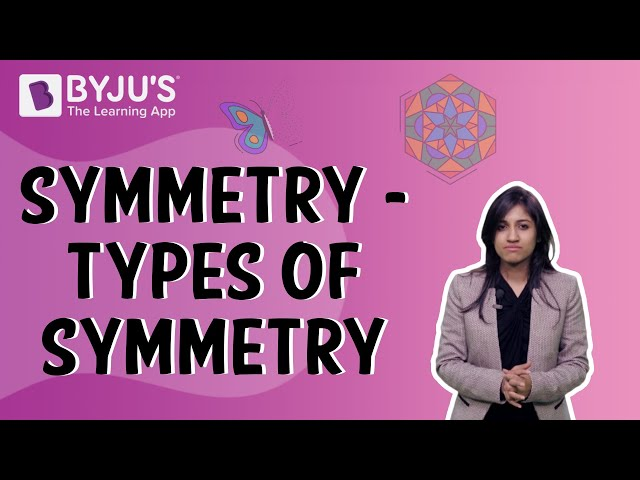Download BYJU’S-The Learning App to learn with the help of personalised videos.

## Frequently Asked Questions on Symmetry – FAQs

### What is symmetry with example?

The word symmetry is the most commonly used concept in the study of reflections of mages. It is often referred to as mirror or reflective symmetry; that means a line or plane that can be drawn through an object such that the two halves are mirror images of each other.

### What are the 4 types of symmetry?

The four types of symmetry are:
Translation symmetry
Rotational symmetry
Reflection (or reflexive) symmetry
Glide symmetry

### What is symmetric in math?

In Mathematics, the meaning of symmetry is that one shape is exactly like the other shape when it is moved, rotated, or flipped.

### Why is symmetry in nature?

Symmetry lies at the heart of the laws of nature. We can observe symmetry in many things that exist in this nature. For example, a line of symmetry can be observed in flowers, butterflies and so on.

### Can a line of symmetry be parallel?

No, not all lines that divide an object into two congruent halves are lines of symmetry. Yet if we try to be creative and draw the line parallel to a set of sides, the folding does not coincide with the shape’s other side. Thus, the line of symmetry cannot be parallel.

1. Titiksha

Cleared all my doubts

2. superb

3. Well done

4. Ishwanshi

This app is best learning app that clears all my doubts

5. it cleared all my doubts

6. Tejaswini Khire

It’s very nice